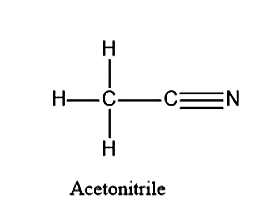QuestionAnswers

# Draw the structure of acetonitrile.Verified
92.1k+ views
Hint:. Acetonitrile is also known as methyl cyanide. It is a chemical compound with the molecular formula $C{H_3}C \equiv N$. We know that carbon will be the central metal atom and Nitrogen and the other carbon atom with methyl group will be attached to it.

Let’s first see what are the elements present in the acetonitrile. Acetonitrile is actually the IUPAC name for methyl cyanide. Acetonitrile is having a formula of $C{H_3}C \equiv N$. From the formula we can see that there are three elements present in the carbon atom. They are C, N and H atoms. There are two carbon atoms present in the acetonitrile.Note: The carbon which is attached to the nitrogen group will have $sp$ hybridization because it is attached to only two atoms namely nitrogen and the carbon of the methyl group. The methyl group carbon will be having a hybridization of $s{p^3}$ because it has been attached to 4 atoms namely, 3 hydrogen atoms and one carbon atom.